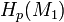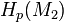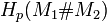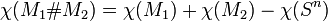# Homology of connected sum

This article describes the effect of the connected sum operation on the following invariant: homology groups

## Statement

Suppose$M_1$ and$M_2$ are the connected manifolds of dimension$n$ whose connected sum is being taken. Assume both We have:

Case for$p$ Additional condition on$M_1,M_2$ What is known about$H_p(M_1)$ and$H_p(M_2)$? Formula for$H_p(M_1 \# M_2)$ in terms of homology groups of$M_1$ and$M_2$
0 none both isomorphic to$\mathbb{Z}$$\mathbb{Z}$ (because both are connected, so is their connected sum).
Greater than 0, less than$n - 1$ none both are finitely generated abelian groups$H_p(M_1) \oplus H_p(M_2)$$n - 1$ Both manifolds are compact, at least one of them is orientable both are finitely generated abelian groups, at least one is free abelian$H_{n-1}(M_1) \oplus H_{n-1}(M_2)$$n-1$ other cases  ?  ?$n$ Both are compact and orientable both are$\mathbb{Z}$$\mathbb{Z}$$n$ other cases  ?  ?
Greater than$n$ none both are zero groups 0

### Euler characteristic

The Euler characteristics are related by the following formula when both$M_1$ and$M_2$ are compact connected manifolds:$\chi(M_1 \# M_2) = \chi(M_1) + \chi(M_2) - \chi(S^n)$